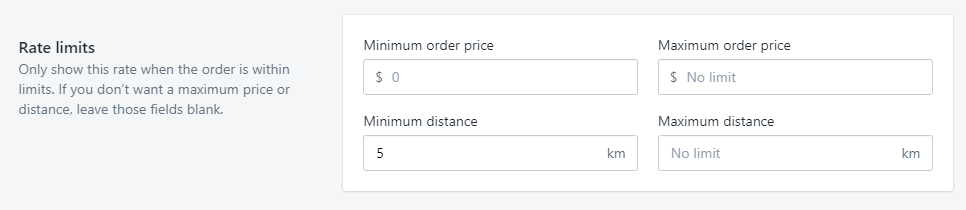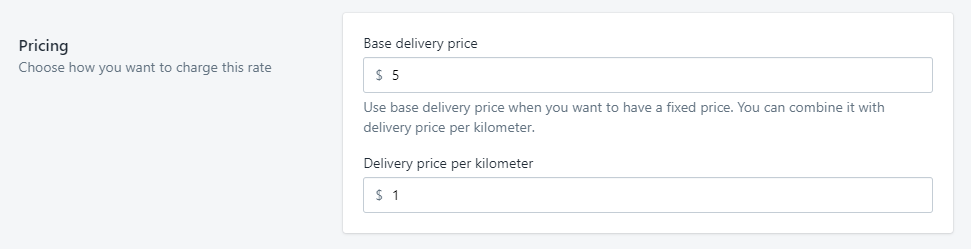# Base fee combined with price per kilometer or mile

Let’s set up the scenario first. We want to

• have a fixed price of \$10 for the first 5 km
• charge \$1 per km after 5 km

To do this, we’ll have to set up 2 rates; one for up to 5 km, and one for distances more than 5 km

For this example we’ll use price-based rates, but you can use weight-based rates too. If you don’t know how to create a rate yet, please check out our create a rate guide.

### Rate 1 - Up to 5 km

1. Let’s give this rate a recognizable name—Delivery up to 5 km.2. For Rate limits, we’ll set

• no minimum order price
• no maximum order price
• no minimum distance
• maximum distance of 5 km3. For Pricing, we’ll set the base delivery fee to \$10, and no price per kilometer.4. Click Create rate

Now that we have the first rate set up, let’s create the next rate.

### Rate 2 - More than 5 km

1. Let’s give this rate a recognizable name—Delivery more than 5 km2. For Rate limits, we’ll set

• no minimum order price
• no maximum order price
• minimum distance of 5 km
• no maximum distance3. For Pricing, we’ll set the base delivery price to \$5, and price per kilometer to \$1.4. Click Create rate.

You should now have these two rates:### Explanation

You might be wondering why we need 2 rates, and why the pricing of rate 2 looks so complicated.

The main thing to remember here is that delivery price per kilometer ignores minimum distance. In other words, delivery price per km always calculates from 0 km, no matter what you put as minimum distance. This means you might need to do a bit of light math to get the base delivery fee for rate 2 set up.

You can use this formula to help you calculate that base delivery fee:

`Base Fee from Rate 1 - (Minimum Distance * Price per Distance) = Base fee for Rate 2`

I know formulas can be hard to understand on their own, so let’s put this one into action.

#### Example 1

For the first example, let’s use the numbers we used at the beginning of this article.

• Base fee (for Rate 1) is \$10 for the first 5 km
• \$1 per km (Price per distance) after 5 km (Minimum distance)
`10 - (5 * 1) = 5`

#### Example 2

For this example, let’s use something straightforward

• Base fee (for Rate 1) is \$5 for the first 5 km
• \$1 per km (Price per distance) after 5 km (Minimum distance)
`5 - (5 * 1) = 0`

#### Example 3

Let’s use bigger numbers this time.

• Base fee (for Rate 1) is \$30 for the first 15 km
• Price per km is \$5 (Price per distance) after 15 km (Minimum distance)
`30 - (15 * 5) = -45`

As you can see here, base delivery fee can indeed go into negatives.

Hope this helped you set up your rates. If not, send us an email to support@zapiet.com and we’ll be happy to help you out.

Did you find it helpful? Yes No

Send feedback